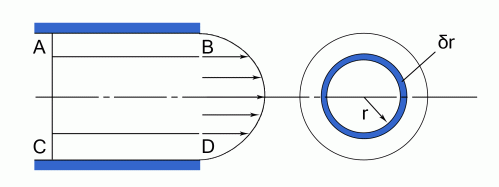•http://facebook.com/
•https://www.google.com/accounts/o8/id
•https://me.yahoo.com

# Pipe Head Loss

The pressure head lost due to flow through pipes and other losses.

## Overview

In general the flow of liquid along a pipe can be determined by the use of The Bernoulli Equation and the Continuity Equation. The former represents the conservation of energy, which in Newtonian fluids is either potential or kinetic energy, and the latter ensures that what goes into one end of a pipe must comes out at the other end. However as the flow moves down the pipe, losses due to friction between the moving liquid and the walls of pipe cause the pressure within the pipe to reduce with distance - this is known as head loss.

Note: Only Incompressible liquids are being considered.

## Head Lost Due To Friction In The Pipe

Two equations can be used when the flow is either Laminar or Turbulent. These are:
##### Darcy's Equation For Round Pipes
For Round Pipes:

where
• is the head loss due to friction [m]
• l is the length of the pipe [m],
• d is the hydraulic diameter of the pipe. For circular sections this equals the internal diameter of the pipe [m].
• v is the velocity within the pipe []
• g is the acceleration due to gravity
• f is the coefficient of friction.

This equation can be expressed in terms of the quantity flowing per second.
##### Darcy's Equation For Non Circular Pipes
where Wetted area / Wetted perimeter
##### The Chezy Equation
where
• i is
• m is or wetted area or wetted perimeter

and
##### Reynolds Number

Which equals 2,300 at the point where the flow changes from Laminar to Turbulent. This is known as the Critical Velocity

If is plotted against

The critical velocity is the velocity at which the change over from laminar to turbulent flow takes place.

Consider a circular pipe running full

Which can be written as The DARCY EQUATION

Note: in the Darcy formula is not an empirical coefficient

For Laminar flow
The change over from laminar flow to turbulent flow occurs when = 2300 and is independent of whether the pipe walls are smooth or rough.

### Laminar Flow

When the flow is laminar it is possible to use the following equation to find the head lost.

The Poiseuille equation states that for a round pipe the head lost due to friction is given by:Flow occurs because the force across is more than that on and hence it is possible to write down the following equation.

Integrating

But when

Thus:

But:

Now the mean velocity is given by:-
From equation (4)
The head lost due to friction is given by:

Substituting from equation (5) for

Example:
[imperial]
##### Example - Example 1
Problem
Water is siphoned out of a tank by means of a bent pipe , 80 ft. long and 1 in. in diameter. is below the water surface and 6 in . above the base sof the tank. is vertical and 30 ft long; is 50 ft. long with the discharge end 5ft. below the base of the tank.

If the barometer is 34 ft. of water and the siphon action at ceases when the absolute pressure is 6 ft. of water, find the depth of water in the tank when the siphon action ceases. is 0.008 and the loss of head at entry to the pipe is:

Where is the velocity of water in the pipe.

Workings
Bernoulli's Equation is:

where Losses
Applying Bernoulli for the pipe length . Note that the pressures quoted in the question have been expressed in ft. of water and therefore:

Applying Bernoulli for the whole pipe length:

From equations (1) and (2)

From which:
Substituting in equation (1)

Solution
The depth of water is

Please note that further worked examples on this topic will be found in the paper on "Hydraulic Gradients"

Last Modified: 24 Feb 12 @ 22:59     Page Rendered: 2022-03-14 15:53:25